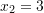### Python Libraries: Sympy

Sympy is a Python library for symbolic computation where variables are represented as symbols. To use Sympy, it must first be imported as follows:

import sympy as sp

## Symbolic Variables in Sympy

To create Symbolic variables that represents a mathematical symbol as opposed to a numeric value, use the symbols() function. This function takes a string of symbolic symbols separated by a space and returns Python variables

x,y = sp.symbols("x y")

To display Sympy expressions, first initialize printing: sp.init_printing() and then use display() to print

To express the following expression in Sympy, use only Sympy variables and Sympy functions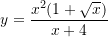import sympy as sp
sp.init_printing()
x = sp.symbols("x")
y = x**2*(1 + sp.sqrt(x))/(x + 4)
display(y)

To substitute numerical values into the symbols, use the <expression>.subs({}) function, which takes a dictionary of the symbols and their numerical values. For example, if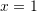,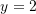, and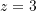, then <expression>.subs({x:1, y:2, z:3})

To evaluate the above expression at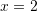, do the following:

import sympy as sp
sp.init_printing()
x = sp.symbols("x")
y = x**2*(1 + sp.sqrt(x))/(x + 4)
y = y.subs({x:2})
display(y)

Notice that Sympy returns the substitution as fractions, to get the decimal equivalent, use the N() function

print(sp.N(y))

## Sympy Matrices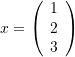To express the above matrix in Sympy, use Matrix():

import sympy as sp
sp.init_printing()
x = sp.Matrix([1,2,3])
display(x)

To express larger matrices like the matrix M below, each row in the matrix would be defined between two square brackets, individual rows are separated by commas and enclosed by a set of square brackets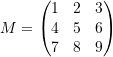import sympy as sp
sp.init_printing()
x = sp.Matrix([[1,2,3],[4,5,6],[7,8,9]])
display(x)

### Matrix Math Operations

Let x and y be two unique vectors, let M be a matrix, and let n be a constant

• Dot Product: x.dot(y)
• Cross Product: x.cross(y)
• Multiplication of a Matrix With a Vector: M*x
• Multiplication of Matrices: M*M
• Scalar Multiplication: n*x
• Addition of Matrices: x+y
• Determinant: M.det()
• Inverse: M.inv()
• Transpose: M.T
import sympy as sp
sp.init_printing()

M = sp.Matrix([[1,2,3],[4,5,6],[7,8,9]])
x = sp.Matrix([1,2,3])
y = sp.Matrix([4,5,6])
display("Dot Product:",x.dot(y))        # 32
display("Cross Product:",x.cross(y))    # [[-3], , [-3]]
display("Multiplication of a Matrix With a Vector:",M*x)
# [, , ]
display("Multiplication of Matrices:",M*M)
# [[30, 36, 42], [66, 81, 96], [102, 126, 150]]
display("Scalar Multiplication:",2*x)   # [, , ]
display("Addition of Matrices:",x+y)    # [, , ]

M = sp.Matrix([[1, 2],[3, 4]])
display("Determinant:",M.det())         # -2
display("Inverse:",M.inv())             # [[-2, 1], [3/2, -1/2]]
display("Transpose:",M.T)               # [[1, 3], [2, 4]]

### Matrix Access Operations

Let M be a matrix, let r be the row number, and let c be the column number

• Get Row r: M.row(r) or M[r,:]
• Get Column c: M.col(c) or M[:,c]
• Element at (r,c): M[r,c]
import sympy as sp
sp.init_printing()

M = sp.Matrix([[1,2,3],[4,5,6],[7,8,9]])
display("M:",M)                     # [[1, 2, 3], [4, 5, 6], [7, 8, 9]]
display("Row 0",M.row(0))           # [[1, 2, 3]]
display("Row 0:",M[0,:])            # [[1, 2, 3]]
display("Col 1",M.col(1))           # [, , ]
display("Col 1:",M[:,1])            # [, , ]
display("Element at (1,2):",M[1,2]) # 6

### Matrix Manipulation Operations

Let M be a matrix, let r be the row number, let c be the column number, and let n be a constant

• Insert to Row r: M = M.row_insert(r,sp.Matrix([[n,...]]))
• Insert to Column c: M = M.col_insert(c,sp.Matrix([n,...]))
• Delete Row r: M.row_del(r)
• Delete Column c: M.col_del(c)
import sympy as sp
sp.init_printing()

M = sp.Matrix([[1,2,3],[4,5,6],[7,8,9]])
display("M:",M)
M = M.row_insert(0, sp.Matrix([[9,9,9]]))
display("Insert to Row 0:",M)
M = M.col_insert(1, sp.Matrix([0,0,0,0]))
display("Insert to Col 1:",M)
M.row_del(0)
display("Delete Row 0:",M)
M.col_del(1)
display("Delete Col 1:",M)

### Sympy Built In Functions

Let x = sp.symbols("x")

• Pi: sp.pi
• Infinity: sp.oo
• Sine: sp.sin(x)
• Cosine: sp.cos(x)
• Log: sp.log(x)
• Exponential: sp.exp(x)
• Factorial: sp.factorial(x)
• Square Root: sp.sqrt(x)
import sympy as sp
sp.init_printing()
x = sp.symbols("x")

display(sp.pi)
display(sp.oo)
display(sp.sin(x))
display(sp.cos(x))
display(sp.log(x))
display(sp.exp(x))
display(sp.factorial(x))
display(sp.sqrt(x))

Let x = sp.symbols("x"), let a and b be the lower and upper bounds, and let n be an integer

• Sum: sp.Sum(x**2,(x,a,b))
• First Derivative: sp.diff(x**2,x)
• Second Derivative: sp.diff(x**2,x,2)
• n-th Derivative: sp.diff(x**2,x,n)
• Integrate: sp.integrate(x**2,(x,a,b))
import sympy as sp
sp.init_printing()
x = sp.symbols("x")

display("Sum:",sp.Sum(x**2,(x,1,2)))
display("First Derivative:",sp.diff(x**2,x))
display("Second Derivative",sp.diff(x**2,x,2))
display("Integrate",sp.integrate(x**2,(x,1,2)))

Let a,b be integers

• Convert to Number: sp.N(a/b)
• Convert to Fraction: sp.Rational(a.b)
• Convert Sympy expression to Numpy function:
• sp.lambdify((symbols), sympy expression)
import sympy as sp
sp.init_printing()

display('N:',sp.N(3/2))
display('Rational:',sp.Rational(1.5))

x, y = sp.symbols('x y')
f = sp.lambdify((x,y), 3*x + 2*y)
display('Sympy to Numpy:', f(1, 2))

### Sum

The built in Sum function can be used to evaluate the sum of a sequence of numbers, consider the following sequence: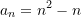Therefore: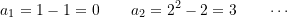To find the sum of the first 10 terms use Sum:

import sympy as sp
sp.init_printing()
n = sp.symbols("n")
display(sp.Sum(n**2 - n, (n,0,10)))
display(sp.N(sp.Sum(n**2 - n, (n,0,10))))

If the infinite sum of the sequence exists, then use the infinity symbol: sp.oo. Consider the following sequence: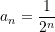Therefore: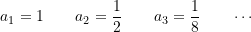import sympy as sp
sp.init_printing()
n = sp.symbols("n")
display(sp.Sum((1/2)**n, (n,0,sp.oo)))
display(sp.N(sp.Sum((1/2)**n, (n,0,sp.oo))))

## Sympy Simplify Expressions

simplify, expand, and factor can be used to simplify a Sympy expression. Let exp be a Sympy expression

• Simplify: sp.simplify(exp)
• Expand: sp.expand(exp)
• Factor: sp.factor(exp)
import sympy as sp
sp.init_printing()
x = sp.symbols("x")

display(sp.simplify(sp.sin(x)**2 + sp.cos(x)**2))
display(sp.expand((x - 2)*(x + 3)))
display(sp.factor(x**2 + x - 6))

## Sympy Solving Equations

Sympy has built-in functions to solve systems of equations. linsolve is used to solve a system of linear equations, nonlinsolve for a system of non-linear equations, dsolve for ordinary differential equations, and nsolve to solve a system of equations numerically

If the solver returns EmptySet() or ∅, then no solution was found, in which case, try another function

### linsolve

sp.linsolve([equations],[variables])

Consider the following linear equation, and find the values of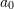,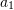, and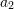that would satisfy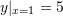,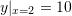, and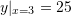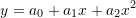Therefore, solve the following system of equations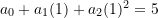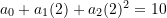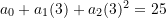import sympy as sp
sp.init_printing()
x, a0, a1, a2 = sp.symbols("x a0 a1 a2")

y = a0 + a1*x + a2*x**2
sol = sp.linsolve([y.subs({x:1}) - 5,
y.subs({x:2}) - 10,
y.subs({x:3}) - 25],
[a0,a1,a2])
display(sol)  # {(10, −10, 5)}
sol = list(sol)
print("a0 = ",sol,"\na1 = ",sol,"\na2 = ",sol)

linsolve returns {(10,-10,5)} which is of type “FiniteSet”. In order to index the solution, convert FiniteSet to list type: sol = list(sol). Then, get the first list inside the outer list using sol to get (10,-10,5). Each element in this list corresponds to each of the variables passed into the solver: (a0,a1,a2). So, a0=sol, a1=sol, and a2=sol

In cases where there are many possible solutions for,, and, Sympy will return all solutions as a separate list in the outer list {(...),(...),...}, so to index the solution to get the n-th solution set use: sol[n]

### nonlinsolve

sp.nonlinsolve([equations],[variables])

Consider the following non-linear equation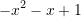import sympy as sp
sp.init_printing()
x = sp.symbols("x")

sol = sp.nonlinsolve([-x**2 - x + 1], [x])
display(sol)
sol = list(sol)
display(sol)
display(sol)

Sympy returns two possible solutions for x: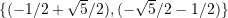. The first solution can be accessed like so: sol and the second like so: sol

### dsolve

Before using dsolve, a Function variable must be defined: y = sp.Function("y"), which is used with respect to a symbol, such as x: y(x)

Initial conditions in dsolve requires Sympy 1.2 or later, update Sympy by running: !pip install sympy --upgrade

sp.dsolve(equation)
# or
sp.dsolve(equation, ics={initial conditions})

Consider the following differential equation: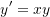import sympy as sp
sp.init_printing()
y = sp.Function("y")
x = sp.symbols("x")

sol = sp.dsolve(y(x).diff(x) - y(x)*x)
display(sol)

Now, solve the constant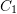using the initial condition,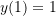:

import sympy as sp
sp.init_printing()
y = sp.Function("y")
x = sp.symbols("x")

sol = sp.dsolve(y(x).diff(x) - y(x)*x, ics={y(1):1})
display(sol)

If dsolve returns an equation: Eq(lhs,rhs), then the actual solution is on the right-hand side: sol.rhs

### nsolve

sp.nsolve([equations],[variables],[initial guesses])

Unlike other solvers, nsolve will return numerical solutions as opposed to symbolic ones. It also requires initial guesses for each of the variables that approximates the answer

Consider the following system of equations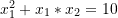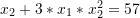import sympy as sp
sp.init_printing()
x1, x2 = sp.symbols("x1 x2")

sol = sp.nsolve([x1**2 + x1*x2 - 10,
x2 + 3*x1*x2**2 - 57],
[x1,x2], [1.5,3.5])
display(sol)

Given initial guesses of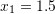and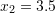, the correct solution is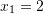and